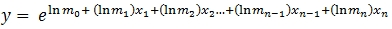# SQL Server predicted values for multivariate exponential regression

EXPPRED
Updated: 02 February 2017
Use the SQL Server scalar function EXPPRED to evaluate a dependent variable from the independent variables using the regression coefficients from the output of the LOGEST or LOGEST_q function. You can use the following equation to calculate the dependent value, y, from the independent variables, x.The m values are included in the output from the XLeratorDB linear regression functions LOGEST and LOGEST_q and have a stat_name of 'm'. The subscripts for m are in the idx column of the regression output.
EXPPRED matches the coefficients of regression (m) to the new independent variables in one of two ways. You can specify that they be matched using the names of the coefficients and the column names of the independent variables or by using the idx associated with m and the relative position of the new independent variables.
Syntax
SELECT [wct].[EXPPRED](
<@Coef, nvarchar(max),>
,<@New_x, nvarchar(max),>
,<@UseNames, bit,>)
Arguments

 Input Name Definition @Coef A string which contains a SELECT statement which returns a resultant table consisting of the idx, stat_val and col_name from the LOGEST or LOGEST_q table-valued functions. @New_x A string which contains either a SELECT statement which returns a single row consisting of the independent variables to be evaluated or a comma separated string of new independent variables. When @New_x is not a SELECT statement @UseNames is ignored and coefficients are match to independent variables based on their position in the string. @UseNames A bit value indicating whether or not use the independent variable names to match up the coefficients with the independent variables. When True the coefficients are match to the independent variables using the names. Then False, the coefficients are match to the independent variables using the column position in the resultant table (or string) returned by @New_x.

Return Type
float
Remarks
·         There will be 1 more coefficient than independent variable
·         When @UseNames is 1, EXPPRED matches the col_name from the regression to the column names in @New_x
·         EXPPRED does not calculate the regression coefficients
·         Available in XLeratorDB / statistics 2008 only
Examples
Example #1
--Put some data in a table
SELECT
IDENTITY(int,1,1) as recno
,*
INTO
#E
FROM (VALUES
(508.78,0.34,3.95,1.75,4.41,49)
,(276.59,0.39,3.72,1.17,3.48,46.72)
,(82.07,0.41,2.35,1.64,3.98,13.03)
,(225.66,0.54,5.08,1.57,3.21,16.32)
,(176.03,0.35,3.31,1.19,3.73,34.16)
,(148.2,0.37,4.46,1.4,4.36,14.62)
,(357.56,0.38,5.51,1.63,3.74,27.97)
,(470.35,0.38,3.74,1.72,3.94,48.8)
,(308.7,0.48,4.76,1.55,3.37,25.84)
,(219.6,0.37,5.03,1.53,4.37,17.6)
)n(y,x0,x1,x2,x3,x4)

--Run the exponential regression and store the results
SELECT
*
INTO
#em
FROM
wct.LOGEST('#E','y,x0,x1,x2,x3,x4','',NULL,1,1)
The regression coefficients are stored in the temp table #em and we can select them using the following SQL.
SELECT
idx
,stat_val
,col_name
FROM
#em
WHERE
stat_name = 'm'
This produces the following result.
As you can see from the resultant table, the regression coefficients can be referenced by the idx column or the col_name column. We can use the EXPPRED function with the @UseNames = 1 and the input data to calculate a predicted y. Note that we have passed all the column name from the input table into the @New_x variable not just the independent variable. By using @UseNames = 1, EXPPRED matched the regression coefficient names to the column names returned by the @New_x SQL.
SELECT
recno
,x1
,x2
,x3
,x4
,y
,wct.EXPPRED(
'SELECT
idx
,stat_val
,col_name
FROM
#em
WHERE
stat_name = ''m'''      --@Coef
,'SELECT
*
FROM
#E
WHERE recno = ' + cast(recno as varchar(max))   --@New_x
,1                         --@UseNames
) as yhat
FROM
#E
This produces the following result.
Example #2
Using the same regression coefficients as in the previous example, we set @UseNames = 0. This means that EXPPRED will not use the regression coefficient names to match to the independent variables but will use the idx values instead which refer to the relative column position in the resultant table from the @New_x SQL. In other words, idx = 1 means the first column, idx = 2 means the second column, etc.
SELECT
recno
,wct.EXPPRED(
'SELECT idx,stat_val FROM #em WHERE stat_name = ''m'''
,'SELECT x0,x1,x2,x3,x4 FROM #E WHERE recno = ' + cast(recno as varchar(max))
,0
) as Yhat
FROM
#E
This produces the following result.
Example #3
Using the same regression coefficients as Example #1 this example shows how to calculate the predicted value when the new x-values are passed in as a comma separated string rather than as a SELECT statement. We use the built-in CONCAT function to build the string a derived table and then pass that value into EXPPRED.
SELECT
wct.EXPPRED(
'SELECT idx,stat_val FROM #em WHERE stat_name = ''m'''
,newx
,0
) as Yhat
FROM (
SELECT
CONCAT(x0,',',x1,',',x2,',',x3,',',x4) as newx
FROM
#E
)n
This produces the following result.

### SupportCopyright 2008-2021 Westclintech LLC         Privacy Policy        Terms of Service